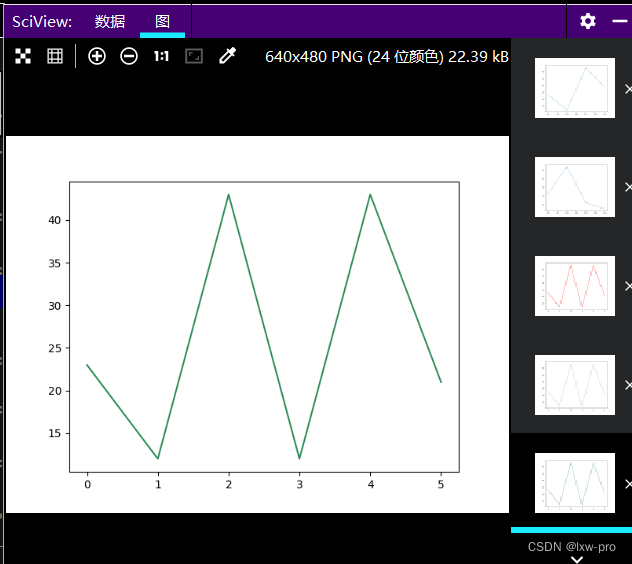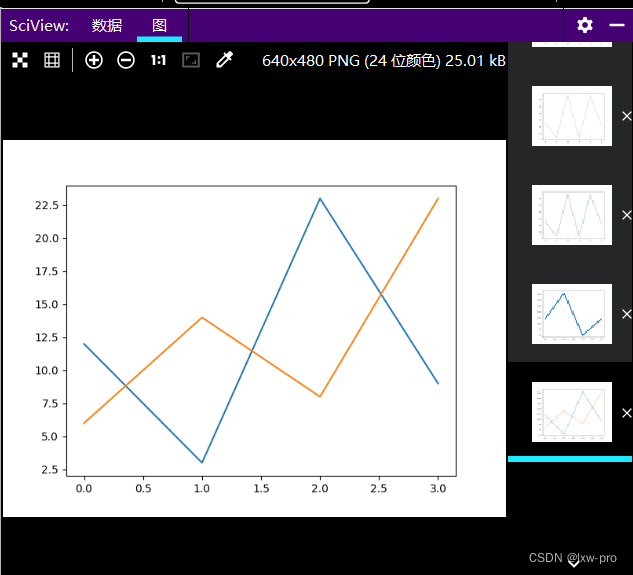# 【数学建模学习笔记【集训十天】之第七天】

### 数模学习目录

• Numpy 学习（续）
• 矩阵运算与线性代数
• 求范数
• 求解线性方程组的唯一解
• 求超定线性方程组的最小二乘解
• 求特殊值及特殊向量
• 运行结果如下：
• SciPy 学习（续）
• SciPy 优化器
• 查找 x + cos(x) 方程的根
• 运行 结果如下：
• 最小化函数
• x^2 + x + 2 使用 BFGS 的最小化函数
• 运行结果如下：
• SciPy Matlab 数组
• 导入 Matlab 格式数据
• Matplotlib 学习（续）
• Matplotlib 绘图线
• 线的类型
• 'dashed'【'–'】 破折线【运用】
• 运行效果如下：
• '-.'【dashdot】 点划线【运用】
• 运行效果如下：
• 线的颜色
• 运行效果如下：
• 运行效果如下：
• 运行效果如下：
• 线的宽度
• 运行效果如下：
• 多条线
• 运行效果如下：
• 更多线
• 运行效果如下：
• 上一章链接：[【数学建模学习笔记【集训十天】之第六天】](https://blog.csdn.net/m0_66318554/article/details/125570128)
• 每日一言：
• 持续更新中…

• # Numpy 学习（续）

## 矩阵运算与线性代数

``````# -*- coding = utf-8 -*-
# @Time : 2022/7/4 9:52
# @Author : lxw_pro
# @File : Numpy库学习-3.py
# @Software : PyCharm

# 矩阵运算与线性代数

# Python中的线性代数运算主要使用numpy.linalg模块，其常用函数如下：
'''
函数              说明
norm            求向量或矩阵的范数
inv             求矩阵的逆阵
pinv            求矩阵的广义逆阵
solve           求解线性方程组
det             求矩阵的行列式
lstsq           最小二乘法求解超定线性方程组
eig             求矩阵的特殊值和特殊向量
eigvals         求矩阵的特殊值
svd             矩阵的奇异值分解
qr              矩阵的QR分解

'''

# 范数计算
'''
计算范数的函数norm的调用格式：
norm(x, ord=None, axis=None, keepdims=False)

参数说明
参数              描述
x       表示要度量的向量或矩阵
ord     表示范数的种类，例如1范数、2范数等
axis：   axis=0表示按列向量处理，求多个列向量的范数；
axis=1表示按行向量处理，求多个行向量的范数
axis=None表示矩阵范数
keepdims    是否保持矩阵的二维特性【True / False】

'''

``````

### 求范数

题目描述

求下列矩阵的各个行向量的2范数，各个列向量的2范数和矩阵2范数``````

import numpy as np
jz = np.array([
[0, 3, 4],
[1, 6, 4]
])                              # 矩阵

h = np.linalg.norm(jz, axis=1)  # 求行向量2范数
l = np.linalg.norm(jz, axis=0)  # 求列向量2范数
f = np.linalg.norm(jz)          # 求矩阵2范数

print(f"行向量2范数为{np.round(h, 4)}")
# 运行结果为：行向量2范数为[5.     7.2801]

print(f"列向量2范数为{np.round(l, 4)}")
# 运行结果为：列向量2范数为[1.     6.7082 5.6569]

print(f"矩阵2范数为{round(f, 4)}")
# 运行结果为：矩阵2范数为8.8318

``````

### 求解线性方程组的唯一解

题目描述：求线性方程组``````import numpy as np
a = np.array([
[3, 1],
[1, 2]
])
b = np.array([9, 8])
# 解法1：
x = np.linalg.inv(a) @ b
print(x)    # 输出 [2. 3.]    表示x=2, y=3

# 解法2：
x2 = np.linalg.solve(a, b)
print(x2)   # 输出 [2. 3.]    表示x=2, y=3

``````

### 求超定线性方程组的最小二乘解

题目描述：求解线性方程组``````import numpy as np
m = np.array([
[3, 1],
[1, 2],
[1, 1]
])
n = np.array([9, 8, 6])
x = np.linalg.pinv(m) @ n
print("最小二乘解为：{}".format(np.round(x, 4)))
# 运行结果为：最小二乘解为：[2.     3.1667]

``````

### 求特殊值及特殊向量

题目描述： 求下列矩阵的特征值和特征向量``````import numpy as np
q = np.eye(4)
w = np.rot90(q)

z, x = np.linalg.eig(w)
print("特征值为：", z)   # 输出  特征值为： [ 1. -1.  1. -1.]
print("特征向量为：\n", x)

``````

#### 运行结果如下：# SciPy 学习（续）

## SciPy 优化器

SciPy 的 optimize 模块提供了常用的最优化算法函数实现，我们可直接调用这些函数完成我们的优化问题

我们可以使用 `SciPy``optimze.root` 函数，这个函数需要两个参数：

• fun – 表示方程的函数
• x0 – 根的初始猜测
• ### 查找 x + cos(x) 方程的根

``````
from scipy.optimize import root
from math import cos

def gen(x):
return x+cos(x)

myroot = root(gen, 0)
print(myroot.x)     # 输出 [-0.73908513]
# 查看更多信息：
print(myroot)
``````

#### 运行 结果如下：### 最小化函数

可以使用 `scipy.optimize.minimize()` 函数来最小化函数

#### x^2 + x + 2 使用 BFGS 的最小化函数

``````
'''
minimize() 函接受以下几个参数：

fun - 要优化的函数

x0 - 初始猜测值

method - 要使用的方法名称，值可以是：'CG'，'BFGS'，'Newton-CG'，'L-BFGS-B'，'TNC'，'COBYLA'，，'SLSQP'。

callback - 每次优化迭代后调用的函数

options - 定义其他参数的字典

'''

# x^2 + x + 2 使用 BFGS 的最小化函数
from scipy.optimize import minimize

def zxh(x):
return x ** 2+x+2

mymin = minimize(zxh, 0, method='BFGS')
print(mymin)

``````
##### 运行结果如下：## SciPy Matlab 数组

NumPy 提供了 Python 可读格式的数据保存方法

``````
'''
SciPy 提供了与 Matlab 的交互的方法
SciPy 的 scipy.io 模块提供了很多函数来处理 Matlab 的数组

以 Matlab 格式导出数据
savemat() 方法可以导出 Matlab 格式的数据。

'''

from scipy import io
import numpy as np

lxw = np.array([5, 2, 0, 1, 3, 1, 4])

io.savemat('lxw.mat', {'vec': lxw})     # 导出

# 注：上面的程序代码会生成一个mat文件

``````

### 导入 Matlab 格式数据

``````# 该方法参数：
# filename - 保存数据的文件名
from scipy import io
import numpy as np

lxw2 = np.array([6, 2, 9, 1, 4, 5])

io.savemat('lxw2.mat', {'vec': lxw2})   # 导出

print(mydata)
# 注：返回一个结构化数组，其键是变量名，对应的值是变量值

# 使用变量名 "vec" 可显示 matlab 数据的数组
print(mydata['vec'])    # 输出 [[6 2 9 1 4 5]]

``````

上面结果变成了二维，须squeeze_me利用降维

``````mydata2 = io.loadmat('lxw2.mat', squeeze_me=True)
print(mydata2['vec'])   # 输出 [6 2 9 1 4 5]
``````

# Matplotlib 学习（续）

## Matplotlib 绘图线

### 线的类型

#### ‘dashed’【‘–’】 破折线【运用】

``````# Matplotlib 绘图线

# 线的类型
# 线的类型可以使用 linestyle 参数来定义【简写为 ls】
'''
类型	            简写	    说明
'solid' (默认)	'-'	    实线
'dotted'	    ':'	    点虚线
'dashed'	    '--'	破折线
'dashdot'	    '-.'	点划线
'None'	    '' 或 ' '	画线

'''

# 'dashed'【'--'】    	破折线
import matplotlib.pyplot as plt
import numpy as np

lx = np.array([23, 12, 43, 29])

plt.plot(lx, linestyle='dashed')

plt.show()

``````
##### 运行效果如下：#### ‘-.’【dashdot】 点划线【运用】

``````# 使用简写:
# '-.'【dashdot】         点划线
from matplotlib import pyplot as plt
import numpy as np

lx2 = np.array([23, 54, 12, 5])

plt.plot(lx2, ls='-.')

plt.show()

``````
##### 运行效果如下：### 线的颜色

红色：

``````# 线的颜色
# 线的颜色可以使用 color 参数来定义，简写为 c
import matplotlib.pyplot as plt
import numpy as np

ys = np.array([23, 12, 43, 12, 43, 21])

plt.plot(ys, color='r')
plt.show()

``````
##### 运行效果如下：绿色

``````# 绿色
plt.plot(ys, c='#8FBC8F')
plt.show()
``````
##### 运行效果如下：深绿色

``````# 深绿色
plt.plot(ys, c='SeaGreen')
plt.show()
``````
##### 运行效果如下：### 线的宽度

``````# 线的宽度
# 线的宽度可以使用 linewidth 参数来定义，简写为 lw
import matplotlib.pyplot as plt
import numpy as np

kd = np.array([12, 23, 5, 12])

plt.plot(kd, linewidth='6.6')
plt.show()

``````
##### 运行效果如下：### 多条线

``````# 多条线
# plot() 方法中可以包含多对 x,y 值来绘制多条线
import matplotlib.pyplot as plt
import numpy as np

d1 = np.array([12, 3, 23, 9])
d2 = np.array([6, 14, 8, 23])

plt.plot(d1)
plt.plot(d2)

plt.show()

``````
##### 运行效果如下：### 更多线

``````import matplotlib.pyplot as plt
import numpy as np

x1 = np.array([1, 2, 3, 6])
y1 = np.array([3, 6, 9, 2])
x2 = np.array([5, 2, 0, 8])
y2 = np.array([6, 2, 9, 3])

plt.plot(x1, y1, x2, y2)
plt.show()

``````
##### 运行效果如下：### 每日一言：

• 有些往事只能回味，眼前的生活要向前看，不是所有的人或事都停留在一瞬，只有经历了才会明白这世界的规则或安定！

•来源：lxw-pro